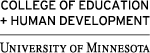EPSY 8269 Matrix Algebra for Statistical ModelingThis course will cover basic concepts of linear and matrix algebra, include examples and applications familiar to students in education and social science research, and include more formal definitions. Topics include vectors and vector operations with applications to statistics, matrices and matrix operations and applications to statistics, and the representation of multivariate statistical models. The class will employ the MATRIX command language available in SPSS. Students may use R or other programs.

Students will deepen their understanding of regression and regression diagnostics from conceptual, computational, and geometric perspectives.

The student will be able to:conduct basic statistical analyses including descriptive statistics and the evaluation of bivariate relationshipsemploy matrix notation to represent multivariate systems of linear equationscompute statistical tests and evaluate multiple systems of equationsuse matrix syntax to conduct common statistical tests

Be sure to check the Class Notes Tab